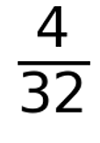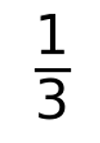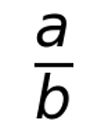# Python | Fraction in Matplotlib

In this article, we are going to learn how to plot a fraction in figure using matplotlib?
Submitted by Anuj Singh, on August 20, 2020

Illustrations:## Python code for fraction in matplotlib

import matplotlib.pyplot as plt

plt.figure()
plt.text(0.5, 0.5, r"$\frac{4}{32}$", fontsize=60)
plt.axis(False)
plt.show()

plt.figure()
plt.text(0.5, 0.5, r"$\frac{1}{3}$", fontsize=60)
plt.axis(False)
plt.show()

plt.figure()
plt.text(0.5, 0.5, r"$\frac{a}{b}$", fontsize=60)
plt.axis(False)
plt.show()


Output:

Output is as Figure